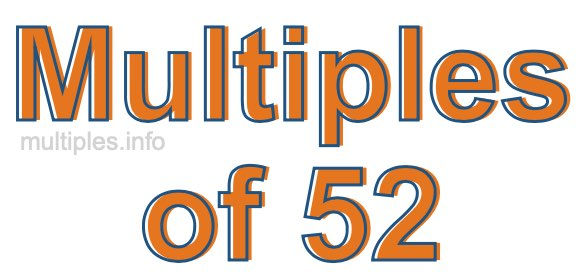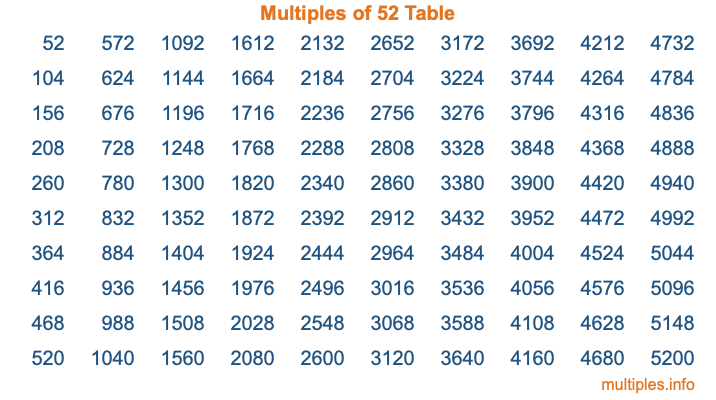Multiples of 52Welcome to the Multiples of 52 page. Here we will first teach you everything you will ever need to know about the multiples of 52, and then give you a study guide summary of everything we taught you to make sure you remember it all. Use this page to look up facts and learn information about the multiples of 52. This page will make you a multiples of fifty-two expert!

Definition of Multiples of 52
Multiples of 52 are all the numbers that when divided by 52 equal an integer. Each of the multiples of 52 are called a multiple. A multiple of 52 is created by multiplying 52 by an integer.

Therefore, to create a list of multiples of 52, you start with 1 multiplied by 52, then 2 multiplied by 52, then 3 multiplied by 52, and so on for as long as you want. Thus, the list of the first five multiples of 52 is 52, 104, 156, 208, and 260. To see a larger list of multiples of 52, see the printable image of Multiples of 52 further down on this page. We also have a category where you can choose any nth multiple of 52.

Multiples of 52 Checker
The Multiples of 52 Checker below checks to see if any number of your choice is a multiple of 52. In other words, it checks to see if there is any number (integer) that when multiplied by 52 will equal your number. To do that, we divide your number by 52. If the the quotient is an integer, then your number is a multiple of 52.

Is  a multiple of 52?

Least Common Multiple of 52 and ...
A Least Common Multiple (LCM) is the lowest multiple that two or more numbers have in common. This is also called the smallest common multiple or lowest common multiple and is useful to know when you are adding our subtracting fractions. Enter one or more numbers below (52 is already entered) to find the LCM.

Check out our LCM Calculator if you need more details about the Least Common Multiple or if you need the LCM for different numbers for adding and subtraction fractions.

nth Multiple of 52
As we stated above, 52 is the first multiple of 52, 104 is the second multiple of 52, 156 is the third multiple of 52, and so on. Enter a number below to find the nth multiple of 52.

th multiple of 52

Multiples of 52 vs Factors of 52
52 is a multiple of 52 and a factor of 52, but that is where the similarities end. All postive multiples of 52 are 52 or greater than 52. All positive factors of 52 are 52 or less than 52.

Below is the beginning list of multiples of 52 and the factors of 52 so you can compare:

Multiples of 52: 52, 104, 156, 208, 260, etc.

Factors of 52: 1, 2, 4, 13, 26, 52

As you can see, the multiples of 52 are all the numbers that you can divide by 52 to get a whole number. The factors of 52, on the other hand, are all the whole numbers that you can multiply by another whole number to get 52.

It's also interesting to note that if a number (x) is a factor of 52, then 52 will also be a multiple of that number (x).

Multiples of 52 vs Divisors of 52
The divisors of 52 are all the integers that 52 can be divided by evenly. Below is a list of the divisors of 52.

Divisors of 52: 1, 2, 4, 13, 26, 52

The interesting thing to note here is that if you take any multiple of 52 and divide it by a divisor of 52, you will see that the quotient is an integer.

Multiples of 52 Table
Below is an image of the first 100 multiples of 52 in a table. The table is in chronological order, column by column. The first column has the first ten multiples of 52, the second column has the next ten multiples of 52, and so on.The Multiples of 52 Table is also referred to as the 52 Times Table or Times Table of 52. You are welcome to print out our table for your studies.

Negative Multiples of 52
Although not often discussed or needed in math, it is worth mentioning that you can make a list of negative multiples of 52 by multiplying 52 by -1, then by -2, then by -3, and so on, to get the following list of negative multiples of 52:

-52, -104, -156, -208, -260, etc.

Multiples of 52 Summary
Below is a summary of important Multiples of 52 facts that we have discussed on this page. To retain the knowledge on this page, we recommend that you read through the summary and explain to yourself or a study partner why they hold true.

There are an infinite number of multiples of 52.

A multiple of 52 divided by 52 will equal a whole number.

52 divided by a factor of 52 equals a divisor of 52.

The nth multiple of 52 is n times 52.

The largest factor of 52 is equal to the first positive multiple of 52.

52 is a multiple of every factor of 52.

52 is a multiple of 52.

A multiple of 52 divided by a divisor of 52 equals an integer.

52 divided by a divisor of 52 equals a factor of 52.

Any integer times 52 will equal a multiple of 52.

Multiples of a Number
Here you can get the multiples of another number, all with the same attention to detail as we did for multiples of 52 on this page.

Multiples of
Multiples of 53
Did you find our page about multiples of fifty-two educational? Do you want more knowledge? Check out the multiples of the next number on our list!

Copyright  |   Privacy Policy  |   Disclaimer  |   Contact